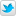# Geometry 2010/11 Lecturer: Prof Salamon

## Exam 27 June 2011Exam 15 July 2011Exam 15 June 2009

Course synopsis
Each module represents at least 12 hours (roughly 2 weeks' material)
"Algebra""Analitica"
Matrices and linear systems
Matrices, sums and products
Powers and inverses of square matrices
Systems of equations, matrix form
Elementary row operations
Row reduction and rank
Gauss(-Jordan) method
Vector analysis
Vectors in space, operations on them
Scalar product, distances, angles
Cross product, triple product, areas, volumes
Plane geometry, lines, conics
Equation of a plane in space
Parametric equation of a line
Rank and dimension
Solving a general system
Row equivalence
Subspaces of Rn
Bases of subspaces
Dimension of subspaces
Spaces generated by rows and columns
Differential calculus
Curves in space, tangent vectors
Arclength, line integrals
Functions with domain in Rn, limits
Continuity, topological notions
Vector-valued functions, Jacobian matrix
Linear mappings
The notion of a vector space
Bases, isomorphic vector spaces
Linear mappings
The matrix of a linear map
Kernel and image, their dimensions
The sum of two subspaces, Grassmann's formula
Functions of two variables
The graph of z=f(x,y), paraboloids
Differentiability, tangent plane
Second partial derivatives, Hessian matrix
Taylor expansion of f(x,y)
Critical points
Extreme values
Diagonalization
Linear transformations, change of basis
Eigenvalues, determinants
Eigenvectors, eigenspaces, multiplicity
Orthogonal matrices and rotations
Diagonalization of square matrices
Surfaces of revolution, spheres
Ellipsoids and hyperboloids
Rotations in Rn
Surfaces defined implicitly
Normal vector, tangent plane

Provisional lecture plan
L = 60 hours and E = 39 hours
14/03LMatrices, sums and products [Summary] Notes, pp 1-4
15/03LPowers and inverses of square matrices [Summary] Notes, pp 5-8

17/03
18/03E
18/03EVectors in space, operations on them Notes, pp 33-34
21/03LSystems of equations, matrix form [Summary] Notes, pp 9-10
22/03LElementary row operations [Summary] Notes, pp 13-14
24/03LGauss(-Jordan) method [Summary] Notes, pp 17-18
25/03E  Notes, pp 10,12,16
25/03EScalar product, distances, angles
Notes, pp 35-36
28/03LRow reduction and rank [Summary] Notes, p 18-19
29/03LSolving a general system [Summary] Notes, pp 21-22
31/03LRow equivalence [Summary] Notes, pp 11,15,20
01/04E  Notes, pp 22-23
01/04ECross product, triple product, areas, volumes Notes, pp 39-40
04/04LSubpsaces of Rn [Summary] Notes, pp 25-26
05/04LBases of subspaces [Summary] Notes, pp 29-30
07/04LDimension and rank [Summary] Notes, pp 31-32
15/04E
15/04ESpaces generated by rows and columns Notes, pp 26-27
11/04LThe notion of a vector space [Summary] Notes, pp 49-50
12/04LMore bases and subspaces [Summary] Notes, pp 51-55
14/04LLinear mappings [Summary]
15/04E
15/04E  Notes, pp 50-51
18/04LBases and linear mappings [Summary] Notes, pp 57-59
19/04LLinear maps, matrices, systems [Summary]
EASTER
28/04L Linear maps, kernels, images [Summary]
02/05L The sum of two subspaces [Summary] Notes, pp 65-66
03/05LEigenvectors and eigenvalues [Summary] Notes, pp 69-71
05/05LEigenspaces [Summary] Notes, pp 77-78
06/05E
06/05EDeterminants
09/05LDiagonalization of square matrices [Summary] Notes, pp 81-82
10/05LDiagonalizing symmetric matrices [Summary] Notes, pp 85-86
12/05LOrthogonal matrices and quadratic forms [Summary]
13/05E
13/05EPlane geometry, conics Notes, p 91 only
16/05LEquation of a plane [Summary] Notes, pp 41-43
17/05LParametric equation of a line [Summary] Notes, pp 45-46
19/05LCurves in space [Summary] MAI, pp279-283
20/05E
20/05E
23/05LLine integrals along curves [Summary] MAI, pp368-375
24/05LFunctions to and from Rn [SUMMARY] MAI, p76, p285
26/05LPartial derivatives, the gradient MAI, p286
27/05E
27/05EGraphs z=f(x,y), paraboloids
30/05LChain rule for scalar functions [SUMMARY] MAI, p288-289
31/05L Scalar functions of 2 and 3 variables [SUMMARY] MAII, p156-162
03/06E
03/06E
06/06LSecond order partial derivatives [SUMMARY] MAII, p168-169
07/06LFirst Taylor expansions [SUMMARY] MAII p161
09/06LCritical points [SUMMARY] MAII, p174-180
09/06EExtreme values (Room 1S at 4pm)
10/06E
10/06E
13/06L Conics and quadrics [SUMMARY] Notes, p 93-95
14/06L Quadrics, conics and lines [SUMMARY]
16/06LExamples and interpretations (at 1000) [Summary]
EModel exam solutions (at 1130)
17/06E
17/06E
20/06LParametrization of surfaces [Summary]
21/06LSurfaces of revolution [Summary]
23/06L Tangents and normals (at 10.00) [Summary corrected]
EWorked sample exercises### References

Note: The summaries should suffice as a basis of theory for the exams !
But more detail is available in the Notes and the following texts (not online).
MAI= Mathematical Analysis I by C. Canuto and A. Tabacco, Universitext, Springer
MAII= Mathematical Analysis II by C. Canuto and A. Tabacco, Universitext, Springer
Elementary Linear Algebra by Howard Anton, 10th edition, Wiley and Sons
Elementary Linear Algebra with supplemental applications by H. Anton and C. Rorres, 10th edition, Wiley, 2010
Updated 08/03/12